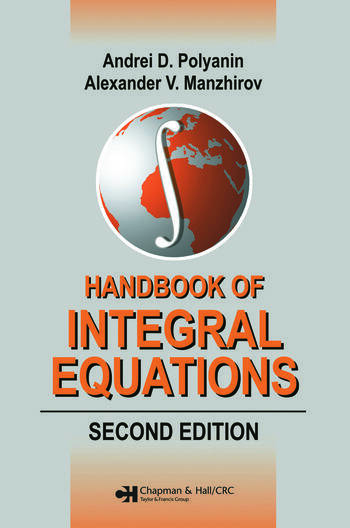# Handbook of Integral Equations: Second Edition

## 2nd Edition

Andrei D. Polyanin, Alexander V. Manzhirov

Chapman and Hall/CRC
Published February 12, 2008
Reference - 1144 Pages - 7 B/W Illustrations
ISBN 9781584885078 - CAT# C5076

USD\$180.00

FREE Standard Shipping!

## Preview

### Summary

Unparalleled in scope compared to the literature currently available, the Handbook of Integral Equations, Second Edition contains over 2,500 integral equations with solutions as well as analytical and numerical methods for solving linear and nonlinear equations. It explores Volterra, Fredholm, Wiener–Hopf, Hammerstein, Uryson, and other equations that arise in mathematics, physics, engineering, the sciences, and economics. With 300 additional pages, this edition covers much more material than its predecessor.

New to the Second Edition

•          New material on Volterra, Fredholm, singular, hypersingular, dual, and nonlinear integral equations, integral transforms, and special functions

•          More than 400 new equations with exact solutions

•          New chapters on mixed multidimensional equations and methods of integral equations for ODEs and PDEs

•          Additional examples for illustrative purposes

To accommodate different mathematical backgrounds, the authors avoid wherever possible the use of special terminology, outline some of the methods in a schematic, simplified manner, and arrange the material in increasing order of complexity. The book can be used as a database of test problems for numerical and approximate methods for solving linear and nonlinear integral equations.